• 正态总体，标准差已知 z=xˉ−μ0σ/nz=\frac{\...某机床厂加工一种零件，根据经验知道，该厂加工零件的椭圆度渐近服从正态分布，其总体均值为0.081mm，今另换一种新机床进行加工，取200各零件进行检验，得到椭圆度均

标准差未知，大样本

z = x ˉ − μ 0 s / n z=\frac{\bar{x}-\mu_0}{s/\sqrt{n}}

• 在总体标准差σ未知时，可以用样本标准差s代替

例：双侧检验

某机床厂加工一种零件，根据经验知道，该厂加工零件的椭圆度渐近服从正态分布，其总体均值为0.081mm，今另换一种新机床进行加工，取200各零件进行检验，得到椭圆度均值为0.076mm，样本标准差为0.025mm，问新机床加工零件的椭圆度总体均值与以前有无差别？

假设：
H 0 : μ = 0.081 m m H_0 : \mu = 0.081mm

H 1 : μ ≠ 0.081 m m H_1 : \mu \neq 0.081mm :

双侧检验

import pandas as pd
import numpy as np
import scipy as sp
from scipy import stats

# 由题可知
n = 200
mu_0 = 0.081
x_bar = 0.076
sigma = 0.025
a = 0.05

计算 z a / 2 z_{a/2}

z_a2 = stats.norm.isf(a/2)
z_a2

计算统计量

z = (x_bar-mu_0)/(sigma/np.sqrt(n))
z

依据统计量 z 决策

if abs(z)<abs(z_a2):
print('不能拒绝H0，差异不显著')
if abs(z)>abs(z_a2):
print('拒绝H0，接受H1')

不能拒绝H0，差异不显著

计算p值

# 计算p值
p_value = stats.norm.cdf(z)*2
p_value

1.8202875051210001

依据p值决策

if p_value > a :
print('不能拒绝H0，差异不显著')

if p_value < a :
print('拒绝H0，接受H1')

不能拒绝H0，差异不显著

例：单侧检验

某电子元件批量生产的质量标准为平均使用寿命1200小时，标准差为150小时。某厂宣称它采用一种新工艺生产的元件质量大大超过规定标准。为了进行验证，随机抽取20件作为样本，测得平均使用寿命为1245小时。能否说该厂的元件质量高于规定标准？

假设：

H 0 : μ ≤ 1200 H_0 : \mu \leq 1200

H 1 : μ > 1200 H_1 : \mu > 1200 :

单侧检验

# 由题可知

mu_0 = 1200
x_bar = 1245
sigma = 150
n = 20

a = 0.05

计算 z a / 2 z_{a/2}

z_a = stats.norm.isf(a)
z_a

计算统计量

z = (x_bar-mu_0)/(sigma/np.sqrt(n))
z

依据统计量z决策

if abs(z)<abs(z_a):
print('不能拒绝H0，差异不显著')
if abs(z)>abs(z_a):
print('拒绝H0，接受H1')

不能拒绝H0，差异不显著

依据p值决策

p_value = stats.norm.sf(z)
p_value

0.08985624743949988

if p_value > a :
print('不能拒绝H0，差异不显著')

if p_value < a :
print('拒绝H0，接受H1')

不能拒绝H0，差异不显著

展开全文python 统计学
• ks检验 特点是比较严格，基于的原理是CDF，理论上可以检验任何分布。 from scipy.stats import kstest --data为数据集，μ为data的均值，δ为data的标准差 kstest(data, 'norm', (μ，δ)) Shapiro检验 专门...

ks检验

特点是比较严格，基于的原理是CDF，理论上可以检验任何分布。

from scipy.stats import kstest

--data为数据集，μ为data的均值，δ为data的标准差
kstest(data, 'norm', (μ，δ))

Shapiro检验

专门用来检验正态分布。

from scipy.stats import shapiro

shapiro(data)

展开全文• Python假设检验

千次阅读 2018-09-26 12:52:22
#假设检验 #前提检验正态分布 observed_temperatures = df['Temperature'].sort_values() bin_val = np.arange(start = observed_temperatures.min(),stop=observed_temperatures.max(),step=50) mu,std = np.mean...
import pandas as pd
import pylab
import math
import numpy as np
import matplotlib.pyplot as plt
%matplotlib inline
from scipy.stats import norm
import scipy.stats
import warnings
warnings.filterwarnings("ignore")

TemperatureGenderHeart Rate
096.3170
196.7171
296.9174
397.0180
497.1173
df.describe()
TemperatureGenderHeart Rate
count130.000000130.000000130.000000
mean98.2492311.50000073.761538
std0.7331830.5019347.062077
min96.3000001.00000057.000000
25%97.8000001.00000069.000000
50%98.3000001.50000074.000000
75%98.7000002.00000079.000000
max100.8000002.00000089.000000
#假设检验
#前提检验正态分布
observed_temperatures = df['Temperature'].sort_values()
bin_val = np.arange(start = observed_temperatures.min(),stop=observed_temperatures.max(),step=50)
mu,std = np.mean(observed_temperatures),np.std(observed_temperatures)

p = norm.pdf(observed_temperatures, mu,std)

plt.hist(observed_temperatures,bins=bin_val,normed=True,stacked=True)
plt.plot(observed_temperatures,p,color='r')
plt.xticks(np.arange(95.75,101.25,0.25),rotation=90)
plt.xlabel('Human Body Temperature Distributions')
plt.ylabel('human body temperature')
plt.show()
print("Average (Mu):"+str(mu)+"/ Standard Deviation:" + str(std))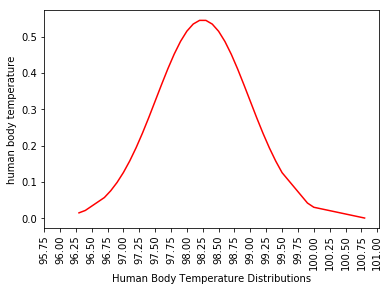Average (Mu):98.24923076923076/ Standard Deviation:0.7303577789050376
#确定指标进行正态检验
x = observed_temperatures
shapiro_test,shapiro_p = scipy.stats.shapiro(x)
print("Shapiro-Wilk Stat:",shapiro_test,"Shapiro-Wilk p-Value:",shapiro_p)

k2,p = scipy.stats.normaltest(observed_temperatures)
print("k2:",k2,"p:",p)

#以上两种方法，p值大于0.05，认为正态分布

#Another method to determining normality is through Quantile-Quantile Plots
#QQ图检查正态分布
scipy.stats.probplot(observed_temperatures,dist='norm',plot=pylab)
pylab.show()
Shapiro-Wilk Stat: 0.9865769743919373 Shapiro-Wilk p-Value: 0.2331680953502655
k2: 2.703801433319236 p: 0.2587479863488212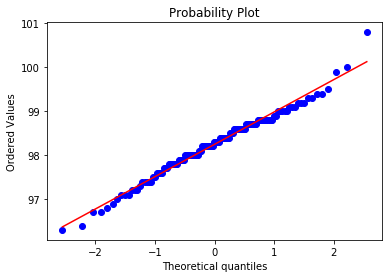#另一种检测正态分布的方法
def ecdf(data):
#Compute ECDF
n = len(data)
x = np.sort(data)
y = np.arange(1,n+1) / n
return x,y
# Compute empirical mean and standard deviation

#Number of samples
n = len(df['Temperature'])

#Sample mean
mu = np.mean(df['Temperature'])

#Sample standard deviation
std = np.std(df['Temperature'])

print("Mean Temperature:",mu,"Standard deviation:",std)

#基于当前的均值和标准差，随机生成一个正态分布
normalized_sample = np.random.normal(mu,std,size=10000)
normalized_x,normalized_y = ecdf(normalized_sample)

x_temperature,y_temperature = ecdf(df['Temperature'])

#Plot the ECDFs
fig = plt.figure(figsize=(8,6))
plt.plot(normalized_x,normalized_y)
plt.plot(x_temperature,y_temperature,marker='.',linestyle='none')
plt.xlabel('ECDF')
plt.ylabel("Temperature")
plt.legend(("Normal Distribution","Sample data"))
Mean Temperature: 98.24923076923076 Standard deviation: 0.730357778905038

Out:

<matplotlib.legend.Legend at 0xb3437b8>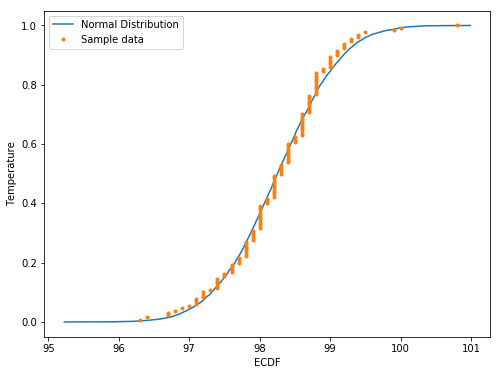#验证98.6为平均温度
from scipy import stats
CW_mu = 98.6
stats.ttest_1samp(df['Temperature'],CW_mu,axis=0)
#T-Stat -5.454 p-value 近乎0，拒绝原假设
Ttest_1sampResult(statistic=-5.454823292364077, pvalue=2.410632041561008e-07)
#检验男女体温是否明显区别
#两独立样本t检验
#H0:两样本没有明显差异，H1:有明显差异
female_temperature = df.Temperature[df.Gender==2]
male_temperature = df.Temperature[df.Gender==1]
mean_female_temperature = female_temperature.mean()
mean_male_temperature = male_temperature.mean()
print("男体温均值：",mean_male_temperature,"女体温均值:",mean_female_temperature)

#两独立样本t检验
stats.ttest_ind(female_temperature,male_temperature,axis=0)

#由于p值0.024 < 0.05 ,拒绝原假设，我们有95%的自信度认为是有差异的
男体温均值： 98.1046153846154 女体温均值: 98.39384615384616

Ttest_indResult(statistic=2.2854345381654984, pvalue=0.02393188312240236)
展开全文• 假设检验实战 1.要解决的问题 给出的数据集为人体的体温数据，下载链接为 https://pan.baidu.com/s/1t4SKF6U2yyjT365FaE692A* 包括三个数据字段： gender：性别，1为男性，2为女性 Temperature:体温 Heart...

1.要解决的问题

给出的数据集为人体的体温数据，下载链接为 https://pan.baidu.com/s/1t4SKF6U2yyjT365FaE692A*

包括三个数据字段：

• gender：性别，1为男性，2为女性
• Temperature:体温
• HeartRate：心率

要解决的问题如下：

• 人体体温的总体均值是否为98.6华氏度？
• 人体的温度是否服从正态分布?
• 人体体温中存在的异常数据是哪些？
• 男女体温是否存在明显差异？
• 体温与心率间的相关性(强？弱？中等?)

首先导入数据集：

import pandas as pd
import numpy as np
import matplotlib.pyplot as plt
import seaborn as sns
from scipy import stats
temp = data['Temperature']
gender = data['Gender']
heartRate = data['HeartRate']

查看该数据集的各项数据

data.describe()

输出：

TemperatureGenderHeartRate
count130.000000130.000000130.000000
mean98.2492311.50000073.761538
std0.7331830.5019347.062077
min96.3000001.00000057.000000
25%97.8000001.00000069.000000
50%98.3000001.50000074.000000
75%98.7000002.00000079.000000
max100.8000002.00000089.000000

输入：

data.shape

输出：

(130, 3)

问题一：人体体温的总体均值是否为98.6华氏度？

这是一个正态总体，方差未知，均值的假设检验问题，使用的是 t 分布

T = X ˉ − μ 0 S / n T=\frac{\bar{X}-\mu_{0}}{S / \sqrt{n}}

∣ T ∣ ≥ t α / 2 ( n − 1 ) |T| \geq t_{\alpha / 2}(n-1)

原假设H0： x ˉ \bar x =98.6

备择假设H1： x ˉ \bar x !=98.6

设置默认显著性水平为 0.05

def hypothesis_mean(data, total_mean, alpha=0.05):
sample_mean = np.mean(data)
sample_std = np.std(data, ddof=1)
sample_size = len(data)
# 检验统计量
t = (total_mean - sample_mean) * np.sqrt(sample_size) / sample_std
t_score = stats.t.isf(alpha / 2, df = (sample_size-1) )
if (t > t_score) or (t < -t_score):
return True
return False

if hypothesis_mean(temp, 98.6):
print('接受H0，人体体温的总体均值为98.6华氏度')

接受H0，人体体温的总体均值为98.6华氏度

问题二：人体的温度是否服从正态分布?

画出热度图，肉眼观察人体温度看是否服从正态分布。

根据中心极限定理，一般情况下，当数据量大于30的时候，可以近似看做服从正态分布，该数据集一共有130条数据，可以近似看做服从正态分布

sns.distplot(temp)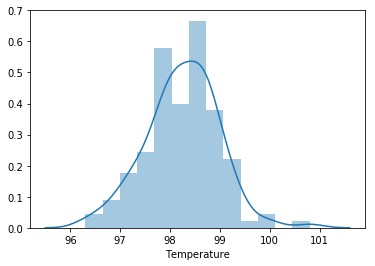下面检验人体的温度是否服从正态分布

scipy.stats.normaltest 可以专门用作检验是否服从正态分布

方法：scipy.stats.normaltest (a, axis=0)

参数

• a - 待检验数据；
• axis - 默认为0，表示在0轴上检验，即对数据的每一行做正态性检验，我们可以设置为 axis=None 来对整个数据做检验

返回

• statistic - 统计量
• pvalue - p值，p大于0.05时，接受原假设H0，即H0为真，p小于0.05时，拒绝H0，即H0为假，接受H1
scipy.stats.normaltest(temp)

输出：

NormaltestResult(statistic=2.703801433319236, pvalue=0.2587479863488212)

根据输出可以看出，p 值为 0.26，大于 0.05，即接受 H0，原假设成立，人体体温服从正态分布

问题三：人体体温中存在的异常数据是哪些？

异常数据，这里采用两种检测方法

1.3σ 原则

3σ 原则又称为拉依达准则，该准则具体来说，就是先假设一组检测数据只含有随机误差，对原始数据进行计算处理得到标准差，然后按一定的概率确定一个区间，认为误差超过这个区间的就属于异常值。

正态分布状况下，数值分布表：

数值分布 在数据中的占比

(μ-σ,μ+σ) 0.6827

(μ-2σ,μ+2σ) 0.9545

(μ-3σ,μ+3σ) 0.9973

注：在正态分布中 σ 代表标准差, μ 代表均值，x=μ 为图形的对称轴

def three_sigma(Ser):
'''
Ser：表示传入DataFrame的某一列。
'''
low = Ser.mean()-3*Ser.std()
up = Ser.mean()+3*Ser.std()
drop_index = Ser.loc[(Ser < low) | (Ser > up)].index
for i in drop_index:
print(Ser[i])
return drop_index, len(drop_index)

three_sigma(temp)

100.8

输出：

(Int64Index(, dtype='int64'), 1)

根据输出，可以看出，使用 3σ 原则检测出来的人体体温中的异常数据只有一个，为 100.8

2.箱线图

箱型图提供了识别异常值的一个标准，即异常值通常被定义为小于 QL-1.5IQR 或大于 QU+1.5IQR 的值

其中，QL 称为下四分位数，表示全部观察值中有四分之一的数据取值比它小；

QU 称为上四分位数，表示全部观察值中有四分之一的数据取值比它大；

IQR 称为四分位数间距，是上四分位数 QU 与下四分位数 QL 之差，其间包含了全部观察值的一半。

# 定义箱线图识别异常值函数
def box_plot(Ser):
'''
Ser：进行异常值分析的DataFrame的某一列
'''
low = Ser.quantile(0.25)-1.5*(Ser.quantile(0.75)-Ser.quantile(0.25))
up = Ser.quantile(0.75)+1.5*(Ser.quantile(0.75)-Ser.quantile(0.25))
drop_index = Ser.loc[(Ser < low) | (Ser > up)].index
return drop_index, len(drop_index)

box_plot(temp)

输出

(Int64Index([0, 65, 129], dtype='int64'), 3)

def view_boxplot(Ser):
plt.subplot(121)
plt.boxplot(Ser)
index, size = box_plot(Ser)
for i in index:
print(Ser[i])

view_boxplot(temp)

96.3
96.4
100.8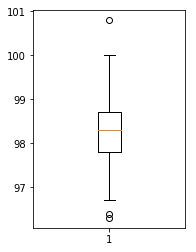根据输出可以看出，使用箱线图检测出来的人体体温中的异常数据有三个，分别为 96.3，96.4，100.8

问题四：男女体温是否存在明显差异？

这是两个正态总体均值差的假设检验问题

由于不确定男女的体温的方差是否相等，因此先用levene检验，检验两总体是否具有方差齐性，若得到的p值大于0.05，认为两总体具有方差齐性。

# levene检验两总体是否具有方差齐性
boy = data['Temperature'][data['Gender'] == 1]
girl = data['Temperature'][data['Gender'] == 2]
stats.levene(boy, girl)

输出：

LeveneResult(statistic=0.06354951292025163, pvalue=0.8013756068102883)

第二个输出为 p 值，可以看出，p 值为 0.80，远大于 0.05，可以认为两总体具有方差齐性。

接下来使用 stats.ttest_ind 函数来检验男女的体温是否存在明显的差异

stats.ttest_ind(boy, girl)

Ttest_indResult(statistic=-2.2854345381654984, pvalue=0.02393188312240236)

得到的 p 值小于 0.05，可以认为拒绝原假设，男女的体温存在明显的差异

问题五：体温与心率间的相关性(强？弱？中等?)

首先可以使用 seaborn 库中的 corr() 函数得到两个变量之间的相关系数，并画出散点图来直观表示

# 相关系数矩阵
temp.corr(heartRate)

0.2536564027207643

# 散点图
plt.scatter(temp, heartRate)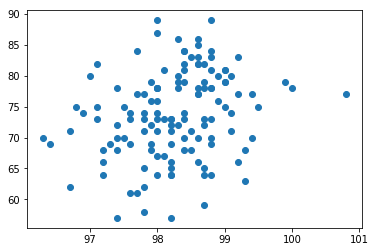相关系数是 0.25， 并且根据图中也可以粗略判断其相关性是不强的

下面介绍两种判断相关系数的方法

1. pearson（皮尔逊）相关系数

要求样本满足正态分布

• 两个变量之间的皮尔逊相关系数定义为两个变量之间的协方差和标准差的商，其值介于-1与1之间

先判断两变量是否服从正态分布

stats.normaltest(temp, axis=0)

输出：

NormaltestResult(statistic=2.703801433319236, pvalue=0.2587479863488212)

stats.normaltest(heartRate, axis=0)

输出：

NormaltestResult(statistic=2.3488941072144778, pvalue=0.3089897872482146)

由于这两个变量的 p 值都大于 0.05，可以认为他们服从正态分布，接下来进行皮尔逊相关系数检验

stats.pearsonr(temp, heartRate)

输出：

(0.25365640272076423, 0.003591489250708233)

p值为 0.03，基本无相关性

2. Sperman 秩相关系数

皮尔森相关系数主要用于服从正太分布的连续变量

对于不服从正太分布的变量，分类关联性可采用 Sperman 秩相关系数，也称等级相关系数

由于本用例给出的变量服从正态分布，所以这里就不再进行 Sperman 秩相关系数检验了

欢迎关注微信公众号 shinerise，与你一起慢慢进步~展开全文假设检验 正态分布 相关性 异常数据 方差检验
• 一项统计结果生成，某市老年人口（65岁以上）所占比例为14.7%，该市老年人口研究会为了检验该项统计是否可靠，随机抽选了400名居民，发现其中由57人年龄在65岁以上，调查记过是否支持该市老年人口比例为14.7%得看法...python 统计学
• python假设检验

2019-11-21 17:54:02
单样本均值Z检验 适合条件：1.总体方差已知，小样本/...=4,所以value=4,alternative[备择假设]='lager' sm.stats.DescrStatsW(moisture['moisture']).ztest_mean(value=4,alternative='lager') 单样本均值t检验 ...
• 利用python进行假设假设检验

千次阅读 2020-08-23 22:12:04
这篇文章通过一个实列来介绍如何利用python来进行假设检验。上一篇文章中，我们介绍了假设检验就是将所要验证的假设分成两个对立的假设，然后验证哪一个假设成立的概率要大一点。python 数据分析
• 如何用python来做假设检验

千次阅读 2020-08-18 23:04:32
其中假设检验包括：t检验，w检验，卡方检验，f检验 ，我将会一一在这篇文章中介绍并用python代码来进行对应实操. (话不多说，整起！) 无论是什么检验第一步永远是 设立假设！ 就像我们初中学的一样已知一个非负数来...数据分析 python
• 利用python进行假设检验

千次阅读 2020-08-17 13:35:51
概念：假设检验，就是要对总体参数u提出一个假设，然后利用样本信息去验证这个假设是否成立。 假设检验的假设： (1)假设检验时，会设置两个假设 一个是原假设，也叫零假设，用H0表示。原假设一般是统计者想要拒绝的...python 数据分析 机器学习
• 假设检验 一、假设概念 假设总体均值为μ，那么实际抽样的均值离μ越近意味着假设越合理，相反，实际抽样均值离μ越远意味着假设越不合理。其中，实际抽样结果与假设的差异“程度”可以用概率值表示，概率值越大意味...python 大数据 数据统计分析 假设检验
• 为什么要写假设检验？因为在工作中经常会遇到ABTest就不可避免的要进行假设检验，进行结果的有效性分析。假设检验的方法有很多比如T检验、卡方检验、方差分析等。这些方法在Excel、Python、R、Spss里都是可以实现的...python做t检验
• 某机器制造出的肥皂厚度为5cm，今欲了解机器性能是否良好，随机抽取10快肥皂作为样本，测得平均厚度为5.3cm，标准差为0.3cm，试以0.05得显著性水平检验机器性能良好得假设 假设： H0:μ=5H_0 : \mu=5H0​:μ=5 H1:μ...python 统计学
• 作者 | Jose Garcia翻译 | 张睿毅校对 | 张一豪来源 | 数据派THU作者给出了假设检验的解读与Python实现的详细的假设检验中的主要操作。也许所有机器...
• 688,632]) 试以a=0.05的显著性水平进行检验 假设： H 0 : μ 1 − μ 2 ≥ 0 H_0:\mu_1 - \mu_2 \geq 0 H0​:μ1​−μ2​≥0 H 1 : μ 1 − μ 2 ​:μ1​−μ2​单侧检验 # 由题可得 n1 = len(A) n2 = len(B) x1_...python 统计学
• 开门见山。这篇文章，教大家用Python实现常用的假设检验！服从什么分布，就用什么区间估计方式，也就就用什么检验！比如：两个样本方差比服从F分布，区间估计就采用F分布计算临界值（从而得出...python 统计学 scipy 机器学习 数据分析
• 假设检验步骤建立原假设H0 和 备假设 H1确定检验统计量确定拒绝区域用样本计算z分数 or t分数 以及 p值接受原假设或者拒绝原假设利用sklearn里的iris数据做假设验证# 导入数据iris = datasets.load_iris()X = iris....
• 我开始学习假设检验时遇到了几个问题： 什么是假设检验？ 为什么要假设检验？ 什么是假设：对总体参数（均值，比例等）的具体数值所作的陈述。（因为一般来说总体的属性具体值我们是测不出来的，也只是靠不停的...数据分析 统计学
• 方差检验 公式 χ2=(n−1)s2σ2\chi^2 = \frac{(n-1)s^2}{\sigma^2}χ2=σ2(n−1)s2​ 例： 某厂商生产出一种新型的饮料装瓶机器，按设计要求，该机器装一瓶1000ml的音量误差上下不超过1ml。如果达到设计要求，表明...python 统计学
• 三、兼谈假设检 假设检验的3种类型 假设检验的套路： 四、利用Python进行相关性分析 判定两者相关的方式有两种： 图形观测法：通过绘制散点图判断两者是否存在一定相关关系 科学计算法：通过计算相关性系数r 我们用...
• 糖厂用自动打包机打包，每包标准重量是100千克。每天开工后需要检验一次打包机工作是否正常。某日开工后测得9包重量(单位：千克)如下：99.3  98.7...用这题演示python实现双边检验的几种方法import numpy as npfro...
• t检验是假设检验中的一种检验方法。t检验可分为单总体检验和双总体检验，以及配对样本检验，主要应用于比较两个平均数的差异是否显著。用t检验的python实现来检测男女生身高和体重是否符合标准。Python实验
• 假设两个正态总体 X∼N(μ1,σ12)，Y∼N(μ2,σ22)X\sim N(\mu_1, \sigma_1^2)， Y\sim N(\mu_2, \sigma_2^2)X∼N(μ1​,σ12​)，Y∼N(μ2​,σ22​). X1,...,Xn1X_1,...,X_{n_1}X1​,...,Xn1​​和 Y1,...,Yn2Y_1,...
• scipy库中stats提供了双侧检验，如果需要单侧检验需要做一下处理 def ttest_onesided(s): from spicy.stats import ttest_1samp (t, p) = ttest_1samp(s, 0) if t > 0: onesided_p = 1 - p / 2 else: ......

python假设检验python 订阅